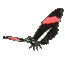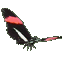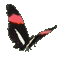Math Help - Improper Fractions & Mixed Numbers - Game Tips:

- The mixed number 'one and two thirds' = (3/3)+(2/3) is equivalent to the improper fraction 5/3.
- The mixed number 'two and a half' = (4/2)+(1/2) is equivalent to the improper fraction 5/2.
- The mixed number 'five and a quarter' = (20/4)+(1/4) is equivalent to the improper fraction 21/4.

- A fraction is called improper when the size of its numerator (top number) is greater than or equal to
the size of its denominator (bottom number).

- Your Game Score is reduced by the number of butterfly hits.

- To slow the game speed repeat tap/click on the word Slider.
- To increase the game speed repeat tap/click on the word Math.
- Speed can also be adjusted with a keyboard's - and + keys.

- Refresh/Reload the web page to restart the game.